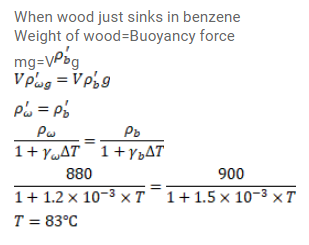# The densities of wood and benzene atQuestion:

The densities of wood and benzene at $0^{\circ} \mathrm{C}$ are $880 \mathrm{~kg} / \mathrm{m}^{3}$ and $900 \mathrm{~kg} / \mathrm{m}^{3}$ respectively. The coefficients of volume expansion are $1.2^{\times 10^{-3} /{ }^{\circ} \mathrm{C}}$ for wood and $1.5^{\times 10^{-3}} /{ }^{\circ} \mathrm{C}$ for benzene. At what temperature will a piece of wood just sink in benzene?

Solution: